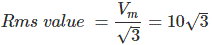# MCQs on Electrical And Electronics Measurements

##### Page 14 of 19. Go to page 1 2 3 4 5 6 7 8 9 10 11 12 13 14 15 16 17 18 19
01․ An average reading digital multimeter reads 10 V when fed a triangular wave, symmetric about the axis. For the same input, an rms reading meter will read
20√3.
10√3.
10.
20.

For triangular wave, average value = Vm/3 Hence Vm = average value X 3 = 10 X 3 = 30 V02․ The effect of stray magnetic fields on the actuating torque of a portable instrument is maximum when the operating field of the instrument and stray fields are
inclined at 30o.
inclined at 60o.
parallel.
perpendicular.

Actuating torque ∝ cos(angle between operating field and magnetic field). For maximum actuating torque, both fields are parallel. (Since cos0o = 1 for maximum value cosine).

03․ Kelvin’s double bridge is used to measure low resistance because
there is no thermo electric emf.
it has high sensitivity.
effect of contact and lead resistance is eliminated.
resistance variation due to temperature.

Effect of lead contact resistance is very important in measurment of low resistance.

04․ To the y input of a CRO a signal defined by 10sin100t is applied. To the x input the signal 10cos100t is fed. The gain for both x-channel and y-channel is the same. The screen shows
a circle.
sinisoidal.
an ellipse.
a straight line.

Here, x2 + y2 = 102 (sin2100t + cos2100t) = 100, which is a constant. This is the equation of a circle. So the screen shows a circle.

05․ An electrodynamic meter can be used to measure
AC volatge.
DC voltage.
both AC and DC voltage.
DC current.

The electrodynamic type meter operates on same principle of series motor. Here one moving coil is placed in between two fixed coils. All these coils are connected in series. The magnetic field created by the fixed coils opposes the magnetic field created by the moving coil. Due to these two opposite magnetic fields, there will be a deflection force acting on the moving coil. The pointer of the meter is attached with moving coil. Hence the pointer moves depending upon the deflecting torque produced in the moving coil. As in the case of series motor, whatever may be the direction of current and whether it is an AC or DC the rotation of the rotor is always unidirectional, the movement of the pointer in electrodynamic meter is always unidirectional irrespective of the direction of current and nature of current.

06․ The main advantage of Anderson bridge over Maxwell bridge is that
reduction of the cost.
balance equation independent of frequency.
attaining balance condition is easier.
measures high Q inductors.

In the Anderson bridge, there is no need of standard variable capacitor to obtain the unknown value of the inductor. We can use normal fixed value capacitor in this bridge. But, we are using the standard variable capacitor in Maxwell bridge. So, the cost of the standard variable capacitor is higher than the normal fixed value capacitor. Hence, the circuit construction cost of Anderson bridge is less than the Maxwell bridge.

07․ The measurement of a quantity
is a comparison of an unknown quantity with known quantity.
is a comparison of an unknown quantity with another quantity.
is a comparison of an unknown quantity with standard.
none of these.

For example, we used to measure the one meter length by one meter scale. Here, one meter scale is accurately known predefined acceptable standard. But, the one meter length is unknown quantity. Here, we are comparing this unknown quantity (one meter length) with the accurately known predefined acceptable standard (one meter scale) and finally we are concluding that the measurement is finished by this process. This is the perfect example of the term measurement.

08․ The internal resistance of Weston standard cell is of order of
1 ohm.
10 ohm.
100 ohm.
1000 ohm.

The internal resistance of an unsaturated Weston standard cell is typically very less. It is normally less than 1 ohm.

09․ The quantity 1/√(∈0 × μ0) in SI units has the
speed of light.
dimension LT-1.
both ‘a’ and ‘b’.
none of these.

0 and μ0 are related as c = 1/√(∈0 μ0); where c is the speed of light.

10․ Which of the following meter is most suitable for measuring radio frequency currents?
Moving - coil meter.
Moving - iron meter.
Thermocouple meter.
VTVM.

In a thermocouple meter, when the current flows through the heating element, the voltage is developed across the open ends of the thermocouple. When the radio frequency current flows through the heating element, it generates more voltage across the open ends. This output voltage is measured by moving coil DC meter. This output voltage is directly proportional to the heat generation in that element. Hence, the thermocouple meter is most suitable for measuring the radio frequency current.

<<<1213141516>>>